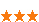您可以捐助，支持我们的公益事业。 1元 10元 50元 认证码：必填求知 文章 文库 Lib 视频 iProcess 课程 认证 咨询 工具 讲座 Modeler Code要资料订阅捐助
python从入门到机器学习982 次浏览     评价： 好 中 差
2018-1-12

 编辑推荐: 来源于csdn，这篇文章主要是讲解了在机器学习的前提下，python应该学习那些内容。

0615

python基本数据结构

1）list: a[1,2,3]

2)tuple: a(1,2,3) 一旦初始化，就不能改变内容

3)dict:{‘a’:1,’b’:2,’c’:3} key:value (dict中的key值不能改变)

4)set: a([1,2,3]) (初始化的时候，传入的是list，set中的key也不能重复，set可以看做是数学意义上的无序和无重复元素的集合)

python的for循环

1)for … in 的循环，一次把list或者tuple中的元素迭代出来

2）for x in …的循环，把每个元素带入变量x中进行计算

python的函数参数

1）默认参数：

 def power(x): -> def power(x,n=2):

2）可变参数：（传入的参数的个数是可变的）

 def calc(numbers): -> def calc(*numbers):

3)关键字参数：

 def person(name,age,**kw):

python的列表生成式

python的列表生成式有点过于强大，能够花式生成各种符合要求的列表，包括全排列，if判断都行。由于内容有点多，这里直接贴一个链接python的列表生成式

python的函数

1）高阶函数：python当中的函数也可以复制给变量

 f = abs cmp(abs(-10),f(-10))#等于0

python当中的map和reduce，还有filter

map(f,nums)意思是将函数f作用于nums这个list中的每一个元素

reduce(f,nums)的作用类似于归约

 def f(s): return s.upper() + s[1:].lower() a = ['adam','batT','LISA'] print map(f,a) #输出位Adam，Batt,Lisa def prod(nums): def fm(x,y): return x*y return reduce(fm,nums) a = [1,3,5] print prod(a) #输出为15，将a中的元素依次相乘 def is_odd(n): return n %2 == 1 filter(is_odd,[1,2,3,4,5]) #输出为[1,3,5] import math def is_prism(n): if n==1: return True if n>2: for i in range(2,int(math.sqrt(n) + 1)): if n %i == 0: return True return False a = range(101) print filter(is_prism,a)

sorted要单独拿出来说，因为它的输入和前面所描述的输入正好相反

sorted(nums,func),sorted可以对list直接排序，但是默认升序，我们可以自定义排序函数来实现sorted函数的任意排序功能

 def reversed_cpm(x,y): if x>y: return -1 if x

python闭包

python中的正则表达式

python中的正则表达式lamda也叫做匿名函数

 def reversed_cpm(x,y): if x>y: return -1 if x

0616

python模块概念

1）

 import sys def test(): args = sys.argv if len(args) == 1: print 'hello' elif len(args)==2: print '2' else: print 'nan' #当此python文件被当作为模块被其他模块引用时，并不会直接执行test函数，if判断将失败。因此这种if测试可以让一个模块通过命令行运行时执行一些额外的代码，最常见的就是运行测试 if __name__=='__main__': test()

2）python，实现函数和变量的私有保护，可以通过_前缀来实现

,_前缀表示该变量/函数为此模块私有，其他模块并不应该访问（不应该访问并不能代表不能访问）

 import sys def _test_(): args = sys.argv if len(args) == 1: print 'hello' elif len(args)==2: print '2' else: print 'nan' def test2(): print 'haha' if __name__=='__main__': _test_()

 import my_sys my_sys.test2() my_sys._test_()

0617

python中的面向对象编程

 class Student(object): def __init__(self,name,score): self.name = name self.score = score def print_info(self): print('%s: %S' % (self.name,self.score)) bart = Student('Bart Simpson',59)

 class Screen(object): @property def width(self): return self._width @property def height(self): return self._height @width.setter def width(self,width): if width<0: raise ValueError('bad value') self._width = width s = Screen() s.width = -1

python定制类

python当中有许多带xxx的函数，这些函数通常是定制函数

 class Fib(object): def __getitem__(self, n): if isinstance(n, int): a, b = 1, 1 for x in range(n): a, b = b, a + b return a if isinstance(n, slice): start = n.start stop = n.stop if start is None: start = 0 a, b = 1, 1 L = [] for x in range(stop): if x >= start: L.append(a) a, b = b, a + b return L f = Fib() print(f) print(f) print(f) print(f[0:5]) print(f[:10])

0618

python文件系统

1)打开和关闭文件

2)操作文件和目录

os.path.abspath(‘.’)#获取绝对路径

os.path.join(‘/usr’,’test’)#合并目录

os.path.split(”)#讲文件名和文件夹名拆分

os.path.splitext()#得到文件的后缀名

 [x for x in os.listdir('.') if os.path.isfile(x) and os.path.splitext(x)=='.py']

 import os def find_files(dir = os.curdir,key = 'python'): adrs=[] for root,folder,files in os.walk(dir): for file in files: tfile = file.partition('.') if key in tfile: adrs.append(os.path.relpath(os.path.join(root,file),dir)) print ('current directin:%s'%os.path.abspath(dir)) print ('find files:%s'%adrs) find_files(key = 't')

python多进程和多线程

linux系统提供了一个fork（）系统调用，它非常特殊。普通的函数调用，调用一次返回一次，但是fork()函数条用一次返回两次。因为操作系统自动吧当前进程（称为父进程）复制了一份（称为子进程），然后分别在父进程和子进程内返回。子进程运用返回0，而父进程返回子进程的ID。这样做的理由是，一个父进程可以fork出很多子进程，所以父进程需要记住每个子进程的ID，而子进程只需要调动getppid()就可以拿到父进程的ID。

 import os print('current process id %s:' % os.getpid()) pid = os.fork() if pid == 0: #child print('child id %s and my father id %s' % (os.getpid(),os.getppid())) else: print('father %s child %s'%(os.getpid(),pid)) #运行结果如下 #current process id 3676: #father 3676 child 3759 #child id 3759 and my father id 3676

 from multiprocessing import Process import os # 子进程要执行的代码 def run_proc(name): print('Run child process %s (%s)...' % (name, os.getpid())) if __name__=='__main__': print('Parent process %s.' % os.getpid()) p = Process(target=run_proc, args=('test',)) print('Child process will start.') p.start() p.join() print('Child process end.' #parent process 928 process will start run child process test(929)... process end

python中的多线程

python当中的正则表达式(re模块)

python基础大概就记了这些笔记，当然python编程是很强大的，分布式和网络编程包括python爬虫等都相当强大。后面写代码的时候，有什么新的发现，我都会及时来更新这篇笔记。982 次浏览  评价: 好 中 差订阅捐助
 相关文章 手机软件测试用例设计实践 手机客户端UI测试分析 iPhone消息推送机制实现与探讨 Android手机开发（一）
 相关文档 Android_UI官方设计教程 手机开发平台介绍 android拍照及上传功能 Android讲义智能手机开发
 相关课程 Android高级移动应用程序 Android系统开发 Android应用开发 手机软件测试